Скачать презентацию From Rainbow to the Lonely Runner Daphne Liu

a961d949e9f132e85bd457ebf099fb08.ppt

• Количество слайдов: 21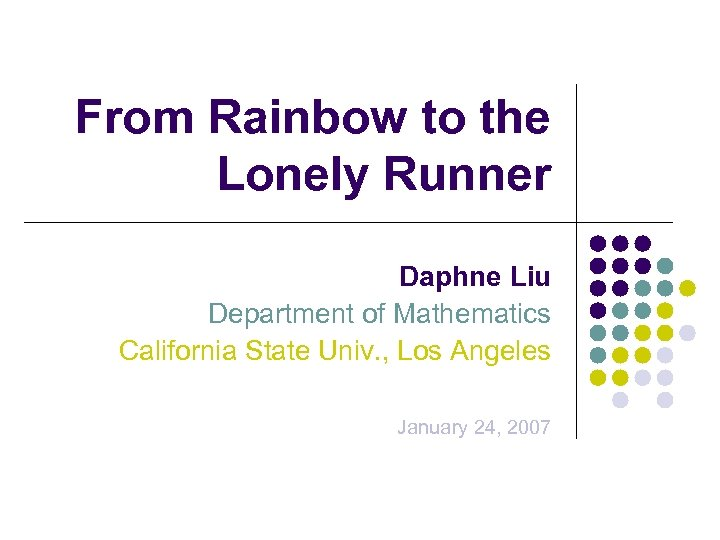From Rainbow to the Lonely Runner Daphne Liu Department of Mathematics California State Univ. , Los Angeles January 24, 2007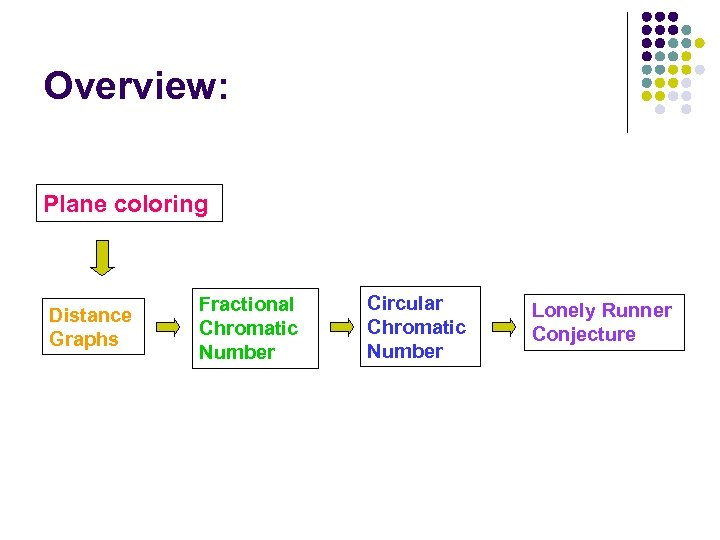Overview: Plane coloring Distance Graphs Fractional Chromatic Number Circular Chromatic Number Lonely Runner Conjecture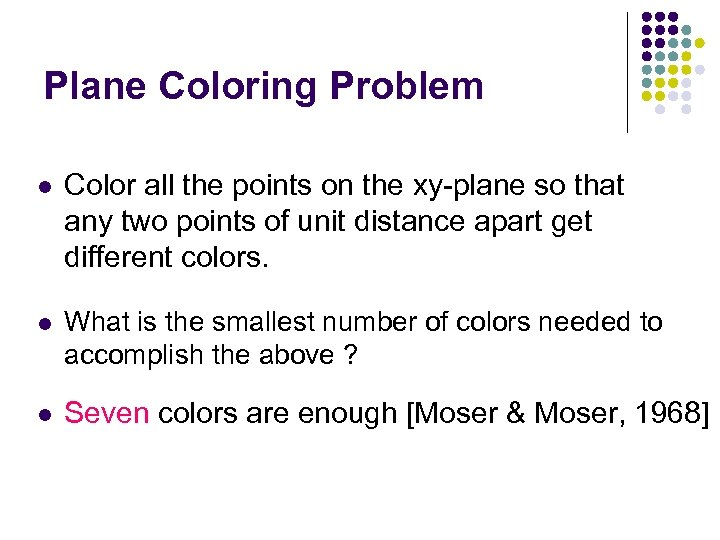Plane Coloring Problem l Color all the points on the xy-plane so that any two points of unit distance apart get different colors. l What is the smallest number of colors needed to accomplish the above ? l Seven colors are enough [Moser & Moser, 1968]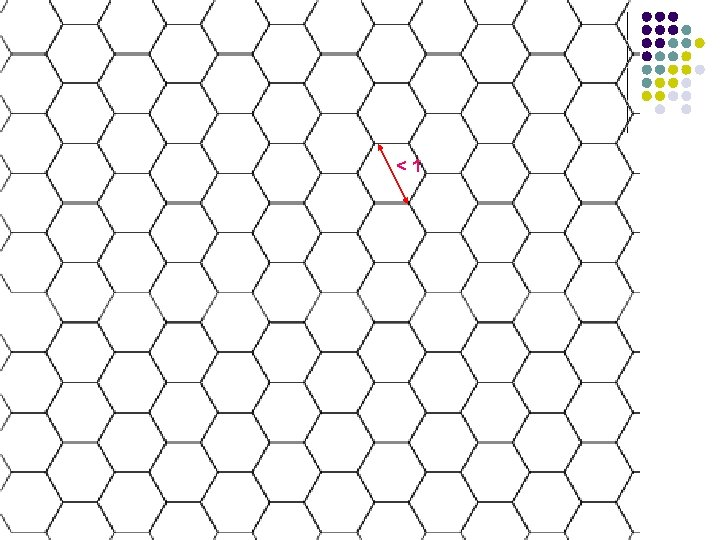<1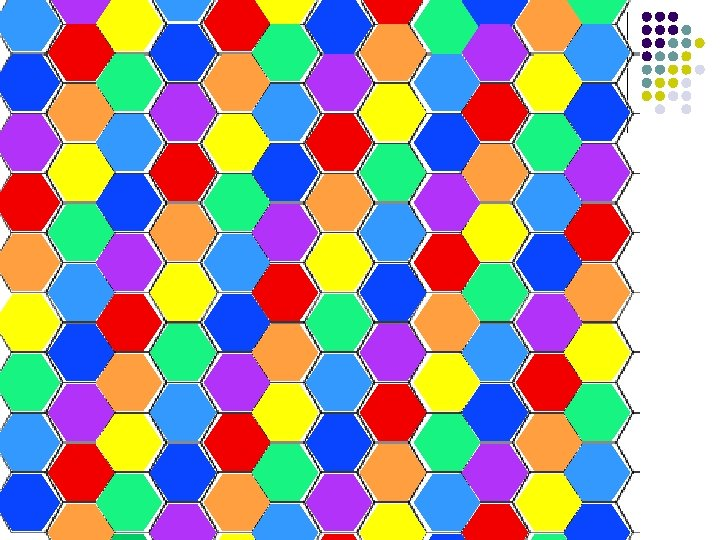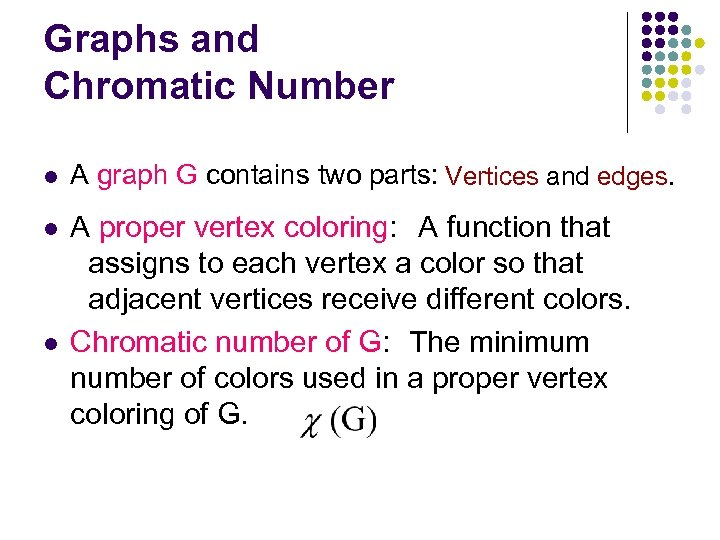Graphs and Chromatic Number l A graph G contains two parts: Vertices and edges. l A proper vertex coloring: A function that assigns to each vertex a color so that adjacent vertices receive different colors. Chromatic number of G: The minimum number of colors used in a proper vertex coloring of G. l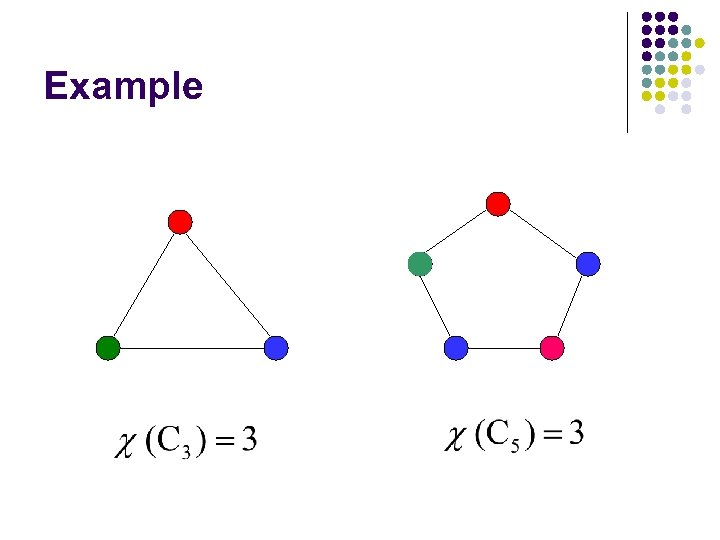Example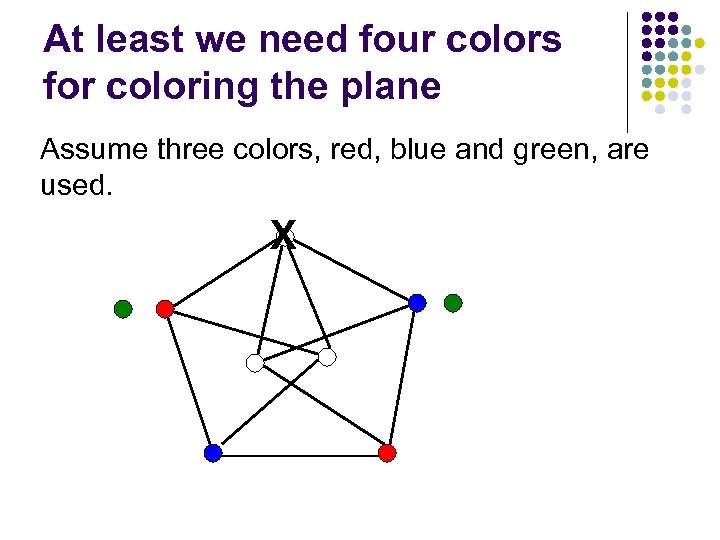At least we need four colors for coloring the plane Assume three colors, red, blue and green, are used. X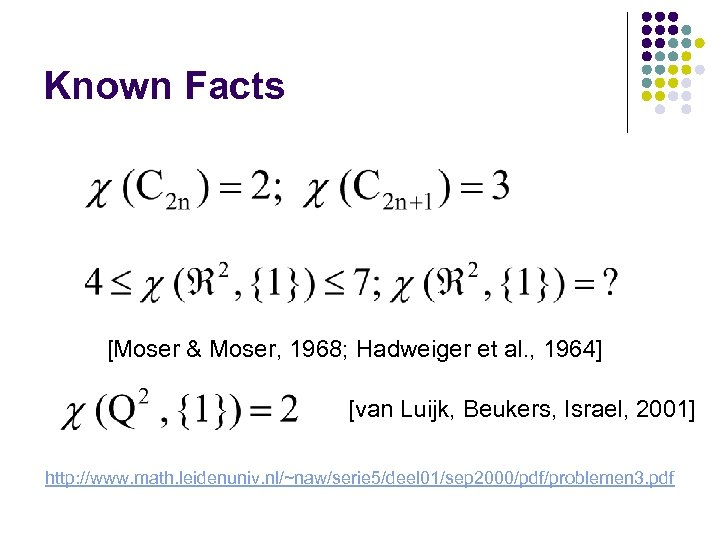Known Facts [Moser & Moser, 1968; Hadweiger et al. , 1964] [van Luijk, Beukers, Israel, 2001] http: //www. math. leidenuniv. nl/~naw/serie 5/deel 01/sep 2000/pdf/problemen 3. pdf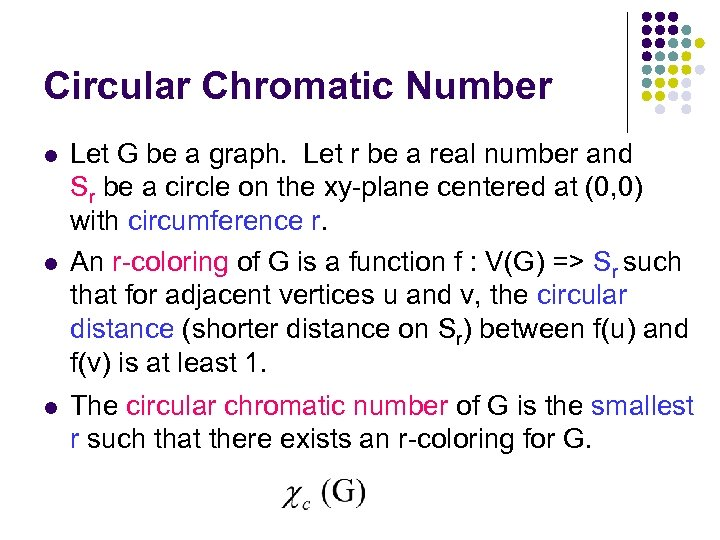Circular Chromatic Number l l l Let G be a graph. Let r be a real number and Sr be a circle on the xy-plane centered at (0, 0) with circumference r. An r-coloring of G is a function f : V(G) => Sr such that for adjacent vertices u and v, the circular distance (shorter distance on Sr) between f(u) and f(v) is at least 1. The circular chromatic number of G is the smallest r such that there exists an r-coloring for G.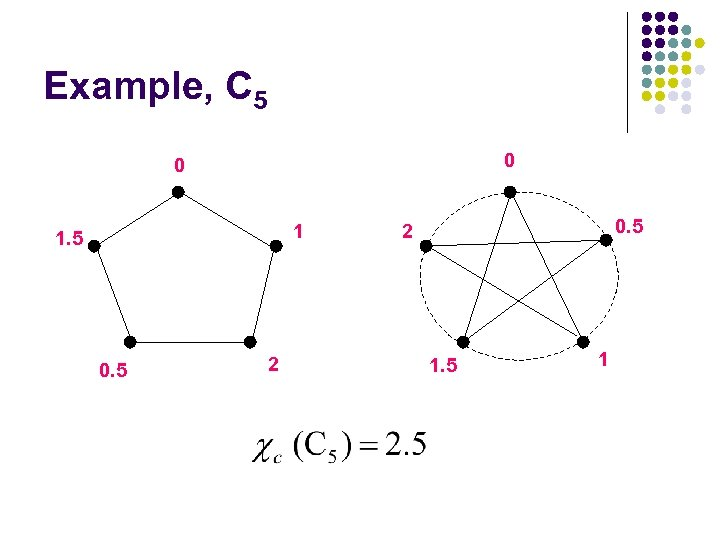Example, C 5 0 0 1 1. 5 0. 5 2 1. 5 1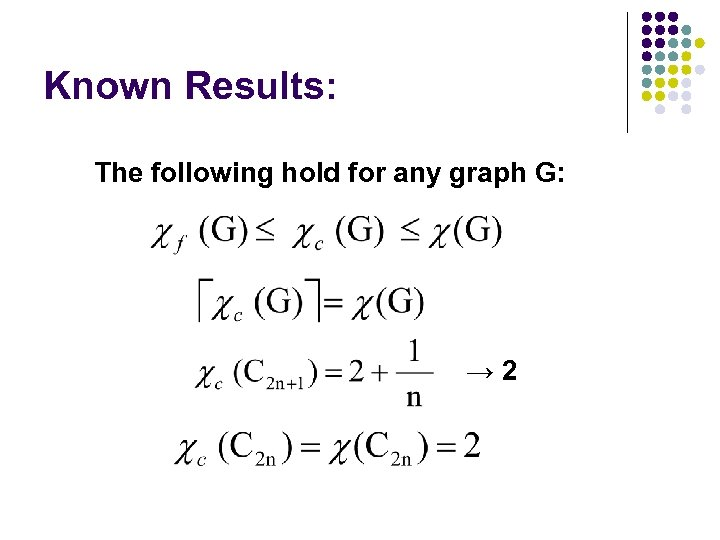Known Results: The following hold for any graph G: → 2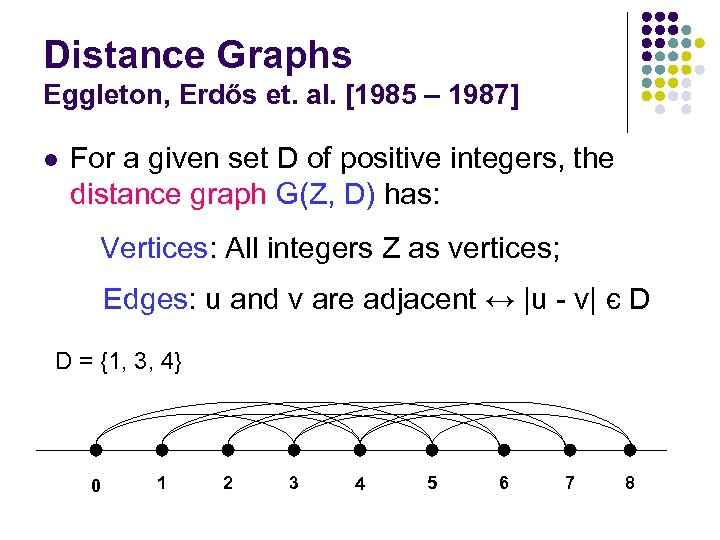Distance Graphs Eggleton, Erdős et. al. [1985 – 1987] l For a given set D of positive integers, the distance graph G(Z, D) has: Vertices: All integers Z as vertices; Edges: u and v are adjacent ↔ |u - v| є D D = {1, 3, 4} 0 1 2 3 4 5 6 7 8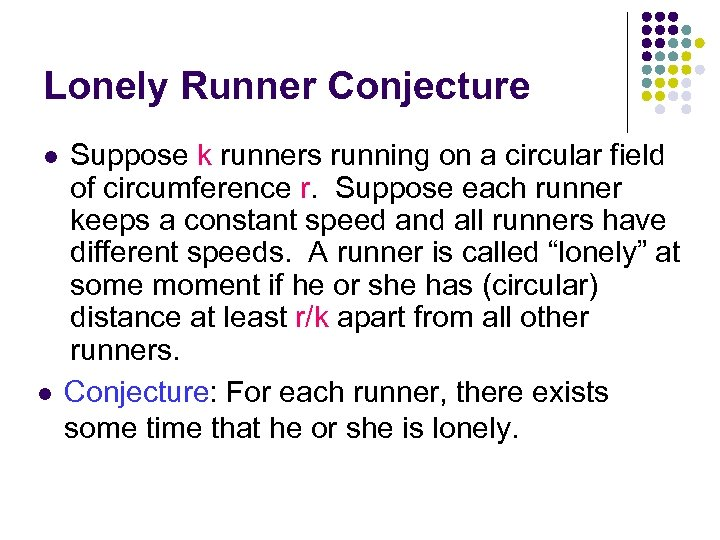Lonely Runner Conjecture l l Suppose k runners running on a circular field of circumference r. Suppose each runner keeps a constant speed and all runners have different speeds. A runner is called “lonely” at some moment if he or she has (circular) distance at least r/k apart from all other runners. Conjecture: For each runner, there exists some time that he or she is lonely.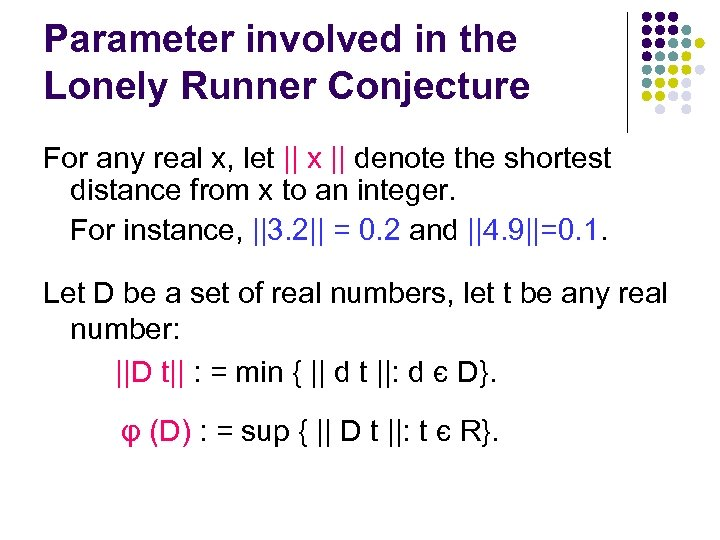Parameter involved in the Lonely Runner Conjecture For any real x, let || x || denote the shortest distance from x to an integer. For instance, ||3. 2|| = 0. 2 and ||4. 9||=0. 1. Let D be a set of real numbers, let t be any real number: ||D t|| : = min { || d t ||: d є D}. φ (D) : = sup { || D t ||: t є R}.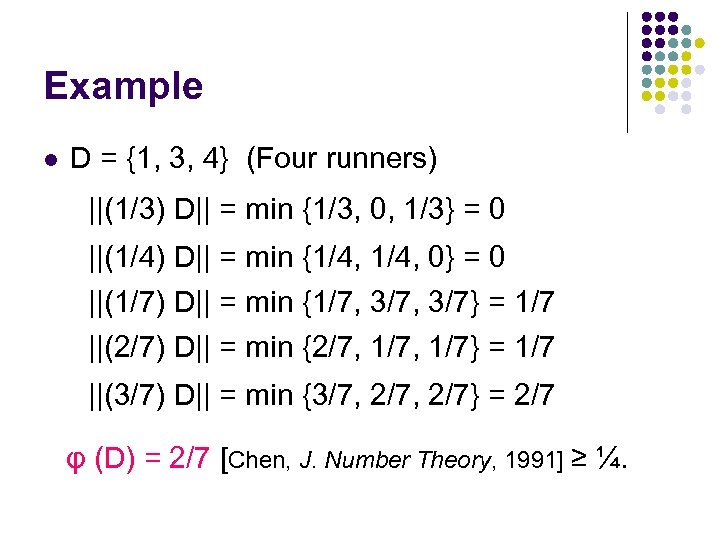Example l D = {1, 3, 4} (Four runners) ||(1/3) D|| = min {1/3, 0, 1/3} = 0 ||(1/4) D|| = min {1/4, 0} = 0 ||(1/7) D|| = min {1/7, 3/7} = 1/7 ||(2/7) D|| = min {2/7, 1/7} = 1/7 ||(3/7) D|| = min {3/7, 2/7} = 2/7 φ (D) = 2/7 [Chen, J. Number Theory, 1991] ≥ ¼.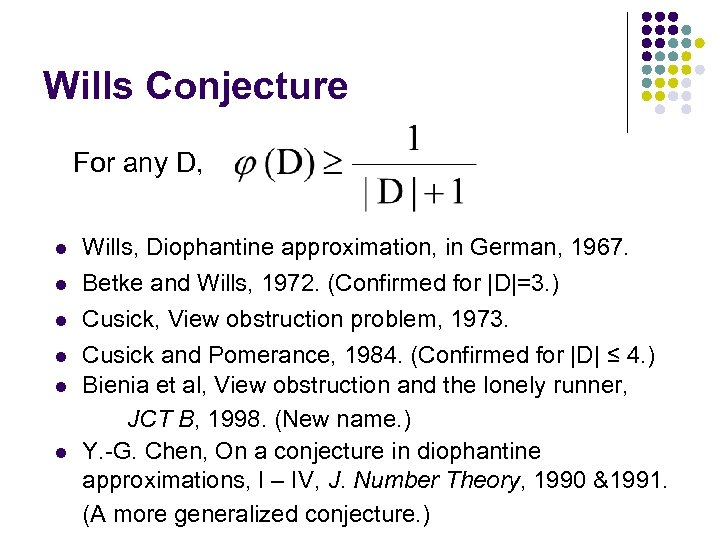Wills Conjecture For any D, l l l Wills, Diophantine approximation, in German, 1967. Betke and Wills, 1972. (Confirmed for |D|=3. ) Cusick, View obstruction problem, 1973. Cusick and Pomerance, 1984. (Confirmed for |D| ≤ 4. ) Bienia et al, View obstruction and the lonely runner, JCT B, 1998. (New name. ) Y. -G. Chen, On a conjecture in diophantine approximations, I – IV, J. Number Theory, 1990 &1991. (A more generalized conjecture. )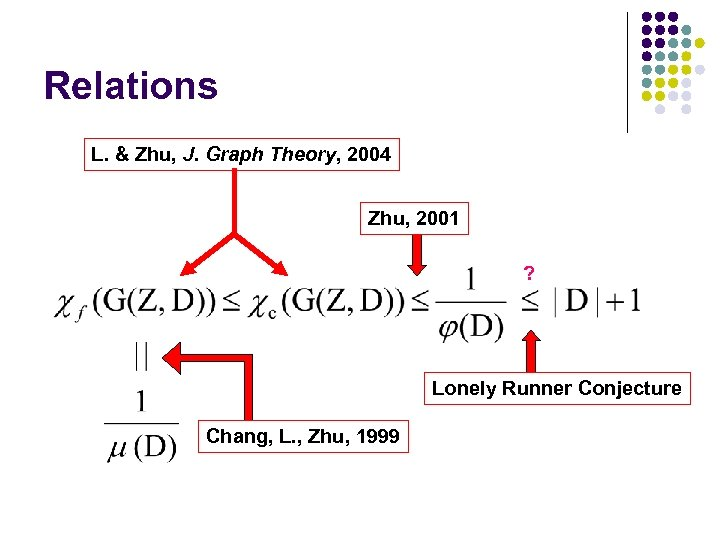Relations L. & Zhu, J. Graph Theory, 2004 Zhu, 2001 ? Lonely Runner Conjecture Chang, L. , Zhu, 1999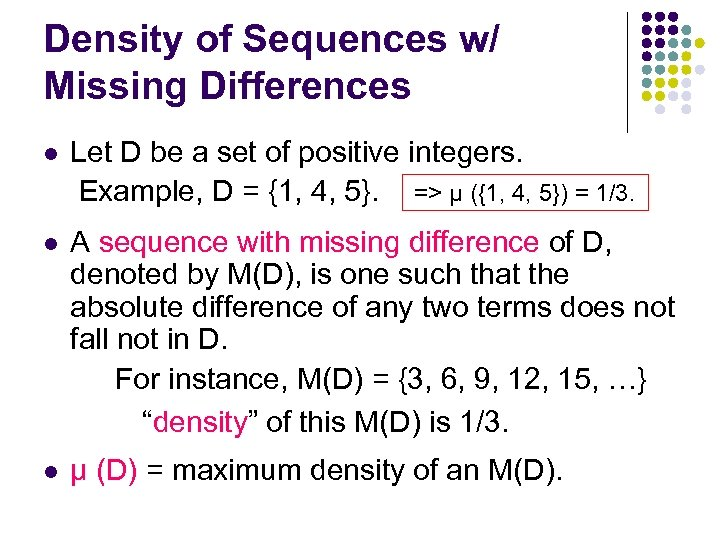Density of Sequences w/ Missing Differences l Let D be a set of positive integers. Example, D = {1, 4, 5}. => μ ({1, 4, 5}) = 1/3. l A sequence with missing difference of D, denoted by M(D), is one such that the absolute difference of any two terms does not fall not in D. For instance, M(D) = {3, 6, 9, 12, 15, …} “density” of this M(D) is 1/3. l μ (D) = maximum density of an M(D).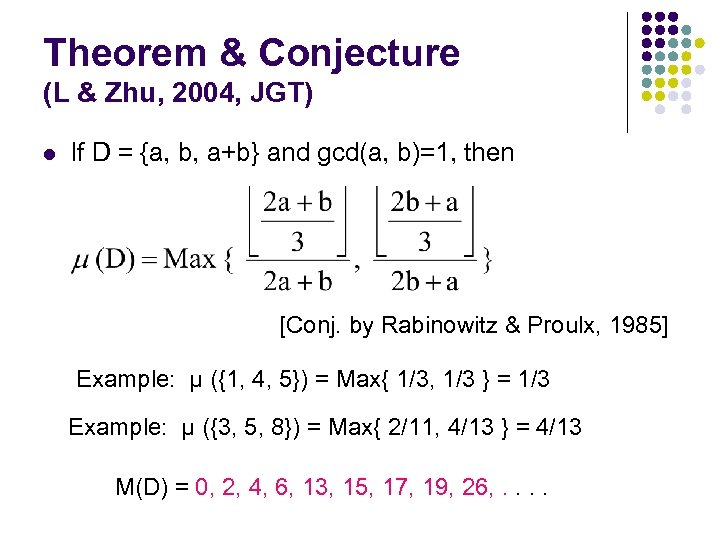Theorem & Conjecture (L & Zhu, 2004, JGT) l If D = {a, b, a+b} and gcd(a, b)=1, then [Conj. by Rabinowitz & Proulx, 1985] Example: μ ({1, 4, 5}) = Max{ 1/3, 1/3 } = 1/3 Example: μ ({3, 5, 8}) = Max{ 2/11, 4/13 } = 4/13 M(D) = 0, 2, 4, 6, 13, 15, 17, 19, 26, . .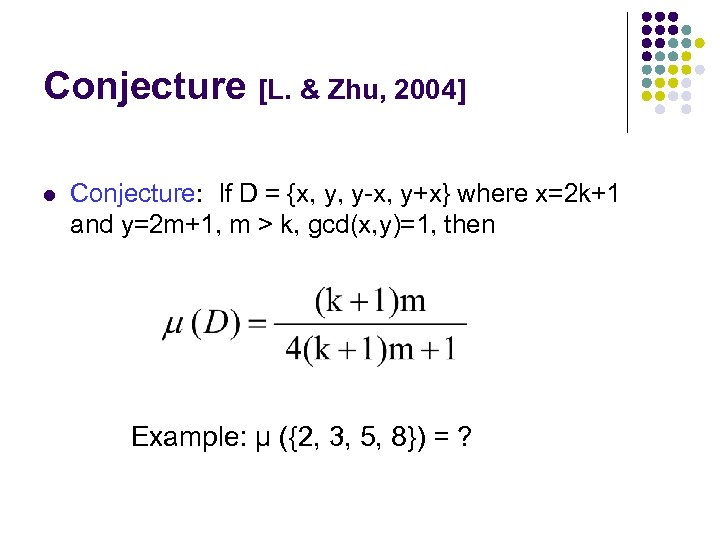Conjecture [L. & Zhu, 2004] l Conjecture: If D = {x, y, y-x, y+x} where x=2 k+1 and y=2 m+1, m > k, gcd(x, y)=1, then Example: μ ({2, 3, 5, 8}) = ?